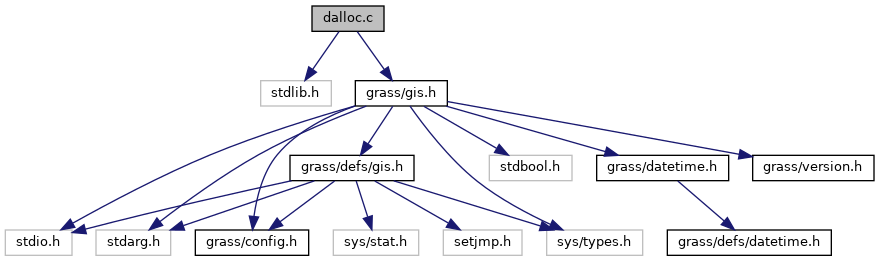GRASS GIS 7 Programmer's Manual  7.9.dev(2021)-e5379bbd7
dalloc.c File Reference

Matrix memory management functions. More...

`#include <stdlib.h>`
`#include <grass/gis.h>`
Include dependency graph for dalloc.c:Go to the source code of this file.

## Functions

double * G_alloc_vector (size_t n)
Vector matrix memory allocation. More...

double ** G_alloc_matrix (int rows, int cols)
Matrix memory allocation. More...

float * G_alloc_fvector (size_t n)
Floating point vector memory allocation. More...

float ** G_alloc_fmatrix (int rows, int cols)
Floating point matrix memory allocation. More...

void G_free_vector (double *v)
Vector memory deallocation. More...

void G_free_fvector (float *v)
Vector memory deallocation. More...

void G_free_matrix (double **m)
Matrix memory deallocation. More...

void G_free_fmatrix (float **m)
Floating point matrix memory deallocation. More...

## Detailed Description

Matrix memory management functions.

This program is free software; you can redistribute it and/or modify it under the terms of the GNU General Public License as published by the Free Software Foundation; either version 2 of the License, or (at your option) any later version.

This program is distributed in the hope that it will be useful, but WITHOUT ANY WARRANTY; without even the implied warranty of MERCHANTABILITY or FITNESS FOR A PARTICULAR PURPOSE. See the GNU General Public License for more details.

You should have received a copy of the GNU General Public License along with this program; if not, write to the Free Software Foundation, Inc., 51 Franklin Street, Fifth Floor, Boston, MA 02110-1301 USA

Date
2004-2006

Definition in file dalloc.c.

## ◆ G_alloc_fmatrix()

 float** G_alloc_fmatrix ( int rows, int cols )

Floating point matrix memory allocation.

Allocate a matrix of rows by cols floats initialized to zero.

Parameters
 [in] rows number of rows in matrix [in] cols number of columns in matrix
Returns
float **

Definition at line 104 of file dalloc.c.

References G_calloc.

## ◆ G_alloc_fvector()

 float* G_alloc_fvector ( size_t n )

Floating point vector memory allocation.

Allocate a vector (array) of n floats initialized to zero.

Parameters
 [in] n size of vector to allocate
Returns
float *

Definition at line 85 of file dalloc.c.

References G_calloc.

## ◆ G_alloc_matrix()

 double** G_alloc_matrix ( int rows, int cols )

Matrix memory allocation.

Allocate a matrix of rows by cols doubles initialized to zero.

Parameters
 [in] rows number of rows in matrix [in] cols number of columns in matrix
Returns
double **

Definition at line 60 of file dalloc.c.

References G_calloc.

## ◆ G_alloc_vector()

 double* G_alloc_vector ( size_t n )

Vector matrix memory allocation.

Allocate a vector (array) of n doubles initialized to zero.

Parameters
 [in] n size of vector to allocate
Returns
double *

Definition at line 41 of file dalloc.c.

References G_calloc.

Referenced by G_math_solver_sparse_gs(), and G_math_solver_sparse_jacobi().

## ◆ G_free_fmatrix()

 void G_free_fmatrix ( float ** m )

Floating point matrix memory deallocation.

Deallocate a matrix of floats.

Parameters
 [in,out] m matrix to free
Returns
void

Definition at line 190 of file dalloc.c.

References G_free(), and NULL.

## ◆ G_free_fvector()

 void G_free_fvector ( float * v )

Vector memory deallocation.

Deallocate a vector (array) of floats.

Parameters
 [in,out] v vector to free
Returns
void

Definition at line 149 of file dalloc.c.

References G_free(), and NULL.

## ◆ G_free_matrix()

 void G_free_matrix ( double ** m )

Matrix memory deallocation.

Deallocate a matrix of doubles.

Parameters
 [in,out] m matrix to free
Returns
void

Definition at line 169 of file dalloc.c.

References G_free(), and NULL.

Referenced by N_free_les().

## ◆ G_free_vector()

 void G_free_vector ( double * v )

Vector memory deallocation.

Deallocate a vector (array) of doubles.

Parameters
 [in,out] v vector to free
Returns
void

Definition at line 129 of file dalloc.c.

References G_free(), and NULL.